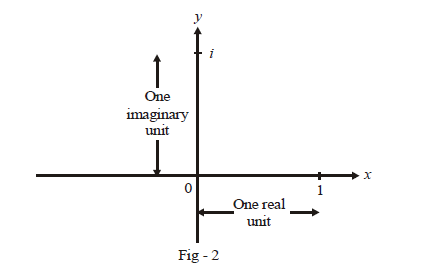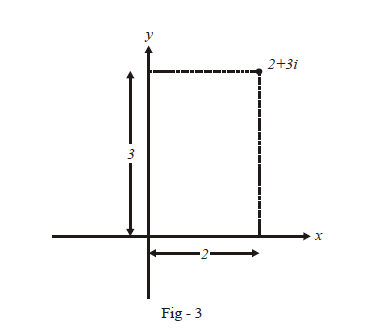# Geometrical Interpretation Of Complex Numbers

Go back to  'Complex Numbers'

Draw the real number line:Since  $$i \notin \mathbb{R},$$ we cannot locate $$i$$ anywhere on this real line. However, what we can do is draw another line perpendicular to the real line, passing through 0, and associate $$i$$ with one unit of length on this new line, which we can term as the imaginary line:What representation would then be given to a point on the imaginary line 5 units above 0?  Answer : $$5i.$$ What about  $$\sqrt 2$$ units below  0? Answer: $$- \sqrt 2 \,i$$ .

Does this make sense? It turns out that this way of associating real numbers with the (horizontal) real line and ‘imaginary’ numbers (of the form $$ki,$$ where $$k$$ is itself real and represents the displacement of that number from 0, on the vertical line) with the (vertical) imaginary line is extremely powerful and useful. A little thought will show you that using this representation, any point on the entire plane can be assigned a unique ‘number’.

For example, the point with co-ordinates (2, 3) can be represented by the ‘number’ $$2 + 3 i.$$This number has both a ‘real’ as well as an ‘imaginary part’.

Similarly, any other point anywhere can be represented uniquely using a single ‘number’ $$x + iy.$$

(The real part, $$x,$$ is nothing but the x-co-ordinate of the point while the imaginary part, $$y,$$ is the y-co-ordinate of the point).

Also, any ‘number’ of the form $$x + iy$$ corresponds to a unique point on the plane.

Thus, we have managed to develop a new number system, consisting of ‘numbers’ of the form $$x + iy,$$ where $$x,y \in \mathbb{R}.$$  We will call such numbers (again due to the convention followed) complex numbers; $$\mathbb{C}$$  is the set of all complex numbers

$\mathbb{C} = \left\{ {x + iy;\,\,\,x,y \in \mathbb{R},\,\,\,\,{i^2} = - 1} \right\}$

Observe that every real numbers $$x \in \mathbb{C}$$  , since x can be written as $$x + i0$$. Therefore, $$\mathbb{C}$$  is a superset of $$\mathbb{R}$$ .

From all this discussion, it does seem after all that $$i$$ is a valid mathematical entity. Associating i with a unit length on the y-axis gives us a number system $$\mathbb{C}$$  capable of representing the entire plane and hence $$\mathbb{C}$$  seems to be a valid ‘improvement’ or extension over $$\mathbb{R}$$  . However it is not just sufficient to define a number system and give it some geometrical significance ourselves. We must also show that the various operations that we use in other number systems, like addition, subtraction, multiplication, and division can also somehow be applied in the set $$\mathbb{C}$$,e complex number system ensures in a consistent manner. Apart from this, we must also show that these operations are consistent with the geometrical significance that we have given to complex numbers.

This is the subject of the next two sections. As a conclusion to this section, you might find it satisfying to know that any polynomial equation of degree $$n$$ is solvable in $$\mathbb{C}$$ , and has $$n$$ roots lying in $$\mathbb{C}$$.

For example:

$$\Rightarrow {x^2} + 1 = 0$$                  has two roots, $$i \;\rm{and}\,– i;$$

both are non-real (complex)

$$\Rightarrow {x^3} + 1 = 0$$                 has three roots, one of them being –1;

the other two are non-real (complex)

Thus, the complex number system ensures the complete solvability of any polynomial equation, which was not possible with just the real number set.

Finally, so that you are clear about it, we mention right here that $$i$$ does exist, in the sense that it has a valid mathematical and physical significance, just as real numbers do. $$i$$ is not just an arbitrary figment of our imaginations  - so in that sense, the term ‘imaginary numbers’ is misleading.

Learn from the best math teachers and top your exams

• Live one on one classroom and doubt clearing
• Practice worksheets in and after class for conceptual clarity
• Personalized curriculum to keep up with school